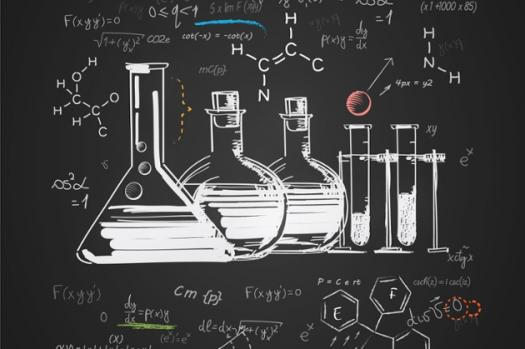# Senior One Quiz No.1

10 Questions | Attempts: 120
ShareSettingsThis quiz is on - systems- specific heat

• 1.
. The property of matter to increase in size on heating is called
• A.

Thermal work

• B.

Thermal energy

• C.

Thermal expansion

• D.

Thermal contraction

• 2.
2. Shining surfaces are
• A.

good absorbers of heat

• B.

• C.

Poor absorbers and poor radiators of heat

• 3.
3. A piece of metal is heated, then submerged in cool water. Which statement below describes what happens?
• A.

a. The temperature of the metal will increase.

• B.

b. The temperature of the water will increase.

• C.

c. The temperature of the water will decrease.

• D.

The temperature of the water will increase and the temperature of the metal will decrease

• 4.
4. How does a calorie compare to a joule?
• A.

a. A calorie is smaller than a joule.

• B.

b. A calorie is larger than a joule.

• C.

c. A calorie is equal to a joule.

• 5.
5. If heat is released by a chemical system, an equal amount of heat will be ____.
• A.

a. absorbed by the surroundings

• B.

b. absorbed by the universe

• C.

c. released by the surroundings

• 6.
-When a thermometer is cooled, the red liquid inside the thermometer moves down. This is mainly because:
• A.

a) Water molecules are warm

• B.

b) Water molecules are in motion

• C.

C) The glass of the thermometer gets cold

• 7.
The quantity of heat required to change the temperature of 1 g of a water by 1 °C is defined as ____.
• A.

Joule

• B.

Calorie

• C.

Specific heat

• 8.
7-How can you describe the specific heat of olive oil if it takes approximately 420 J of heat to raise the temperature of 7 g of olive oil by 30°C?
• A.

a. greater than the specific heat of water

• B.

b. less than the specific heat of water

• C.

C. equal to the specific heat of water

• 9.
How many joules are in 148 calories? (1 cal = 4.18 J)
• A.

619

• B.

6.61

• C.

3

• 10.
If 25 J are required to change the temperature of 5.0 g of substance A by 2.0°C, what is the specific heat of substance A?
• A.

2.5 J/g°C

• B.

250 J/g°C

• C.

1 j/g c

## Related TopicsBack to top
×

Wait!
Here's an interesting quiz for you.